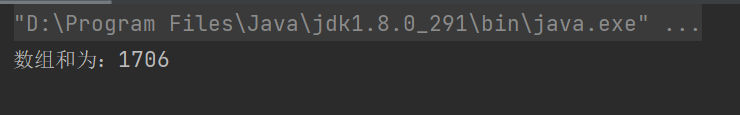• Java:一维数组求和,最大最小,平均值!
2021-10-27 09:12:04

#问题:
定义一个int型的一维数组，包含10个元素，分别赋 一些随机整数，然后求出所有元素的最大值，最小值，总和，平均值，并输出出来。

代码:

package mojiaibiancheng;

public class Demo6 {

public static void main(String[] args) {
// TODO Auto-generated method stub
int[] a = new int;
for(int i = 0 ; i < a.length ; i++) {
a[i] = (int)(Math.random()*100);
}
int max = a , min = a , sum = 0;
for(int i = 0 ; i < a.length ; i++) {
if(a[i] > max) {
max = a[i];
}
}
for(int i = 0 ; i < a.length ; i++) {
if(a[i] < min) {
min = a[i];
}
}
for(int i = 0 ; i < a.length ; i++) {
sum += a[i];
}
System.out.println("最大值:"+max);
System.out.println("最小值:"+min);
System.out.println("最和:"+sum);
System.out.println("平均值:"+sum/10.0);

}
}开发语言 后端
更多相关内容
• ## java一维数组求和

千次阅读 2021-03-13 05:48:52
publicclassHello{publicstaticvoidmain(String[]args){//建立个50元素组成的数组把1-100的所有偶数放在数组里并显示int[]a=newint;intj=2;for(inti=0;ia[i]=j;j+=2;}System.out.println("该数组所有元素为：...

import java.util.*;

public class Hello {

public static void main(String[] args) {

//建立一个50元素组成的数组 把1-100的所有偶数放在数组里 并显示

int[]a=new int;

int j=2;

for(int i=0;i

a[i]=j;

j+=2;

}

System.out.println("该数组所有元素为：");

for(int x:a){

System.out.print(x+" ");

}

System.out.println();

// 为上述数组里 前10个单元求和

int sum=0;

for(int i=0;i<10;i++){

sum+=a[i];

}

System.out.println("该数组前十个数之和为："+sum);

//求上述数组前20个单元的平均数

int vge=0;

int sum1=0;

for(int i=0;i<20;i++){

sum1+=a[i];

}

vge=sum1/20;

System.out.println("数组前20个单元的平均数:"+vge);

//将上述数组第1单元和第21单元对调，第2单元和第22单元对调，第3单元和第23单元对调,

//依次类推，直到第10单元和第30单元对调，并显示数组全元素

for(int i=0;i<10;i++){

int temp;

for(int j1=20;j1<30;j1++){

temp=a[i];

a[i]=a[j1];

a[j1]=temp;

}

}

System.out.println("新数组元素为：");

for(int x:a){

System.out.print(x+" ");

}

System.out.println();

//将上述数组中，所有能被5整除的元素+3。并显示数组全元素

for(int i=0;i

if(a[i]%5==0){

a[i]+=3;

}

}

System.out.println("新数组元素为：");

for(int x:a){

System.out.print(x+" ");

}

System.out.println();

//求上述数组第6单元到第40单元里面 最大数 和最小数

int max=a;

int low=a;

for(int i=5;i<40;i++){

if(a[i-1]>max){

max=a[i-1];

}

if(a[i-1]

low=a[i-1];

}

}

System.out.println("第6单元到第40单元里面 最大数:"+max);

System.out.println("第6单元到第40单元里面 最小数:"+low);

//将该数组从大到小排序。并显示数组全元素

Arrays.sort(a);

System.out.println("排序后的数组为：");

for(int i=a.length-1;i>=0;i--){

System.out.print(a[i]+" ");

}

}

}

展开全文• ## JAVA一维数组求和

千次阅读 2019-11-06 17:47:32
public class Main { public static void main(String[] args) { int num[]={1,2,3,4,5,6,7,8,9,10}; //定义一维数组 int sum = 0; System.out.println("一维数组中个元素之和为："); for(int ...

本网所有内容文字、图片和音视频资料，版权均属个人所有，仅供个人呢学习参考，任何媒体、网站或个人未经本网协议授权不得转载、链接、转贴或以其他方式复制发布/发表。

public class Main {
public static void main(String[] args) {
int num[]={1,2,3,4,5,6,7,8,9,10};				//定义一维数组
int sum = 0;
System.out.println("一维数组中个元素之和为：");
for(int i=0;i<num.length;i++){
System.out.print(num[i]);
if (i==9) {
System.out.print("=");            }
else if (i<9){
System.out.print("+");
}
sum=sum+num[i];
}
System.out.print(sum);
}
}

展开全文• 1.小马猿之前呢已经把还有保存的C++语言的代码分享给了伙伴们，接下来就开始进入Java语言的使用了。在这呢小马猿先分享几个实用的...2.一维数组求和代码 public class arraySum { public static void main(String

1.小马猿之前呢已经把还有保存的C++语言的代码分享给了伙伴们，接下来就开始进入Java语言的使用了。在这呢小马猿先分享几个实用的快捷键。

（1）psvm+回车，在class中可快速生成main方法。

（2）sout+回车，在class中可快速生成输出语句

（3）Ctrl+d,赋值当行到下一行

（4）定量后+ .for + 回车可快速生成for循环。

2.一维数组求和代码

public class arraySum {
public static void main(String[] args) {
int sum = 0;
int[] arr = {68,27,95,88,171,996,51,210};//任意创建一个一维数组
for(int i=0;i<arr.length;i++){//用数组长度进行循环，并用sum记录叠加
sum += arr[i];
}
System.out.println("数组和为：" + sum);
}
}


3.结果：小小代码奉上，希望有所帮助。

展开全文开发语言
• 本教程操作环境：windows7系统、java10版，DELL G3电脑。1.数组求和public class Main {public static void main(String[] ... //定义一维数组int sum = 0;System.out.println("一维数组中个元素之和为：");for(in...
• // 计算数组元素中的平均值 public static int avg(int arr[]){ int N=arr.length; int sum=0; for(int i=0;i<N;i++){ sum+=arr[i]; } int average=sum/N; return average; } 在主方法中调用方法 ...算法
• 维数组-求和 遍历二维数组，并将各个值累计到 int sum public class TwoDimensionalArray05 { //编写个main方法 public static void main(String[] args) { /* int arr[][]={{4,6},{1,4,5,7},{-2}}; ...leetcode 排序算法
• //定义了一个长度为3，即三个一维数组，这三个一维数组的长度都为4 int[][]nums = new int[];//这种定义方法只定义了二维数组的长度，没有确定每个数组的元素个数 int[][]nums ={{30,35},{11,23,13,24},{25,...
• 1，二维数组求和 /* 需求：公司年销售额求和 某公司按照季度和月份统计的数据如下：单位(万元) 第季度：22,66,44 第二季度：77,33,88 第三季度：25,45,65 第四季度：11,66,99 */ class Test2_Array { ...
• ## Java二维数组列求和

千次阅读 2019-07-10 20:33:00
/定义个205的二维数组，用来存储某班级20位学员的5门课的成绩；这5门课 按存储顺序依次为：core C++，coreJava，Servlet，JSP和EJB。 （1）循环给二维数组的每个元素赋0~100之间的随机整数。 （2）按照列表的...
• 一维数组遍历与求和：public class OneArry{public static void main(String[] args) {double[] num = {1.9, 2.9, 3.4, 3.5,10,-1};num=new Double(2); //通过下标把1.9变为2.0double sum = 0;for (int i = 0; i ...
• 杨辉三角是一个由数字排列成的三角形数表，一般形式如下：11 11 2 11 ...通过一维数组来实现：重点放在“更新两个字上”第一次打印时让第一个元素为1第二次打印时让最后一个元素为1，第一个元素不变第三次打印时，让...
• 给定个二维数组，请你编写求和函数，计算出这个数组元素的和 输入描述： 无 输出描述： 输出二维数组元素的和 示例1 输入： 无 输出： 5180 public class Main { public static void main...
•算法 分治算法 c#
• ## 数组求和统计

千次阅读 2021-03-14 18:03:35
## 代码中的类名、方法名、参数名已经指定，请勿修改，直接返回方法规定的值即可## 计算有多少对符合条件的数对# @param a int整型一维数组 数组a# @param b int整型一维数组 数组b# @return int整型#class Solution...
• 一维数组1.一维数组的定义方式：int[] array1 = new int;//声明创建一个包含3个元素的数组array1(初始值为0)int[] array2 = {1, 2, 3};//声明、创建并初始化一个包含3个元素的数组int[] array3 = new int[] {1, 2...
• ClassWork02.java1 package lesson05;23 /**4 * 编程计算一维数组的最大值、最小值、总和、平均值与标准差5 */678 public class ClassWork02 {9 public static void main(String[] args) {10 int[] data = ne...
• 数组知识点什么是数组我们知道...当我们在创建数组时，会在内存中划分出块连续的内存用于存储数据，插入数据时，会将数据按顺序存储在这块连续的内存中，读取时通过访问数组的索引迅速取出。数组名就是个指针，...
• 1.创建数组 大致结构为 数据类型 数组名字=new 数据类型[数组大小] 有两种写法（没有什不同 ，只是在内存分配的先后） int list1[ ]=new int;//声明和分配同时进行 int list2[];//先声明在分配 list2=new int...
• 一维数组 1.一维数组的定义方式： int[] array1 = new int;//声明创建一个包含3个元素的数组array1(初始值为0) int[] array2 = {1, 2, 3};//声明、创建并初始化一个包含3个元素的数组 int[] array3 = new int[] {...
•数据结构 stream 排序
• 记录学习 import java.util.Scanner; public class JW5_1{ public static void main(String ar[]){ testArray(); } public static void testArray() { int[][] array = new int;//创建二维数组 ...算法
• 定义个二维数组，用于存储个3*3矩阵元素值，并求出该矩阵对角线元素之和，最后进行输出 public class test1 { /** * 定义个二维数组，求出该矩阵对角线元素之和 */ public static void main(String[] args...
• 一维数组 定义格式： //格式1：数据类型[] 数组名； int[] a； //定义了一个int类型的数组a； //格式2：数据类型 数组名[]; int a[]; //定义了一个int类型的a数组； //推荐使用第一种定义方式......

# java一维数组求和java 订阅